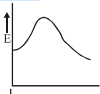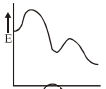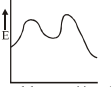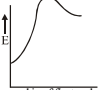# NEET Chemistry Chemical Kinetics Questions Solved

The solvolysis of 2-chloro-2-methyl propane in aqueous acetone: ${H}_{2}O+{\left(C{H}_{3}\right)}_{3}C-Cl\to HO-C{\left(C{H}_{3}\right)}_{3}+{H}^{+}C{l}^{-}$ has a rate equation.

Rate =From this it may be inferred that the energy profile of the reaction leading from reactants to products is

(A)(B)(C)(D)Concept Videos :-

#8 | Problems on 1st Order Reaction (Type 1)
#9 | Problems on 1st Order Reaction (Type 2)
#10 | Problems on 1st Order Reaction (Type 3)
#12 | Problems on 1st Order Reaction (Type 5)

Concept Questions :-

First Order Reaction Kinetics